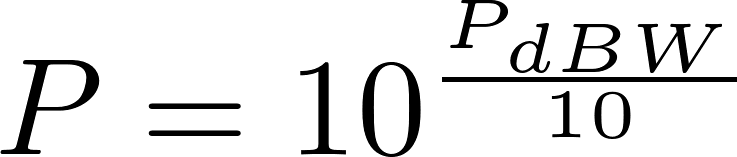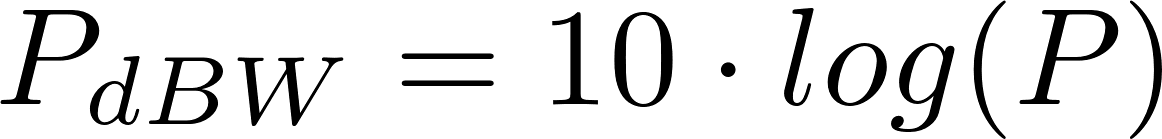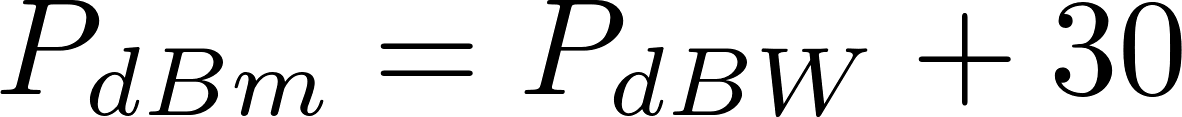# Decibel (dBW)

The decibel (dB) is used to express the ratio of two values on a logarithmic scale. The decibel watt (dBW) is the unit for the measurement of the strength of a signal expressed in decibels relative to one watt. It is very useful as it can express a large range of values in a short range of numbers.

### FormulasP is the symbol for power and is measured in watt (W).
P dBW is the symbol for decibel watt.
P dBm is the symbol for decibel milliwatt.